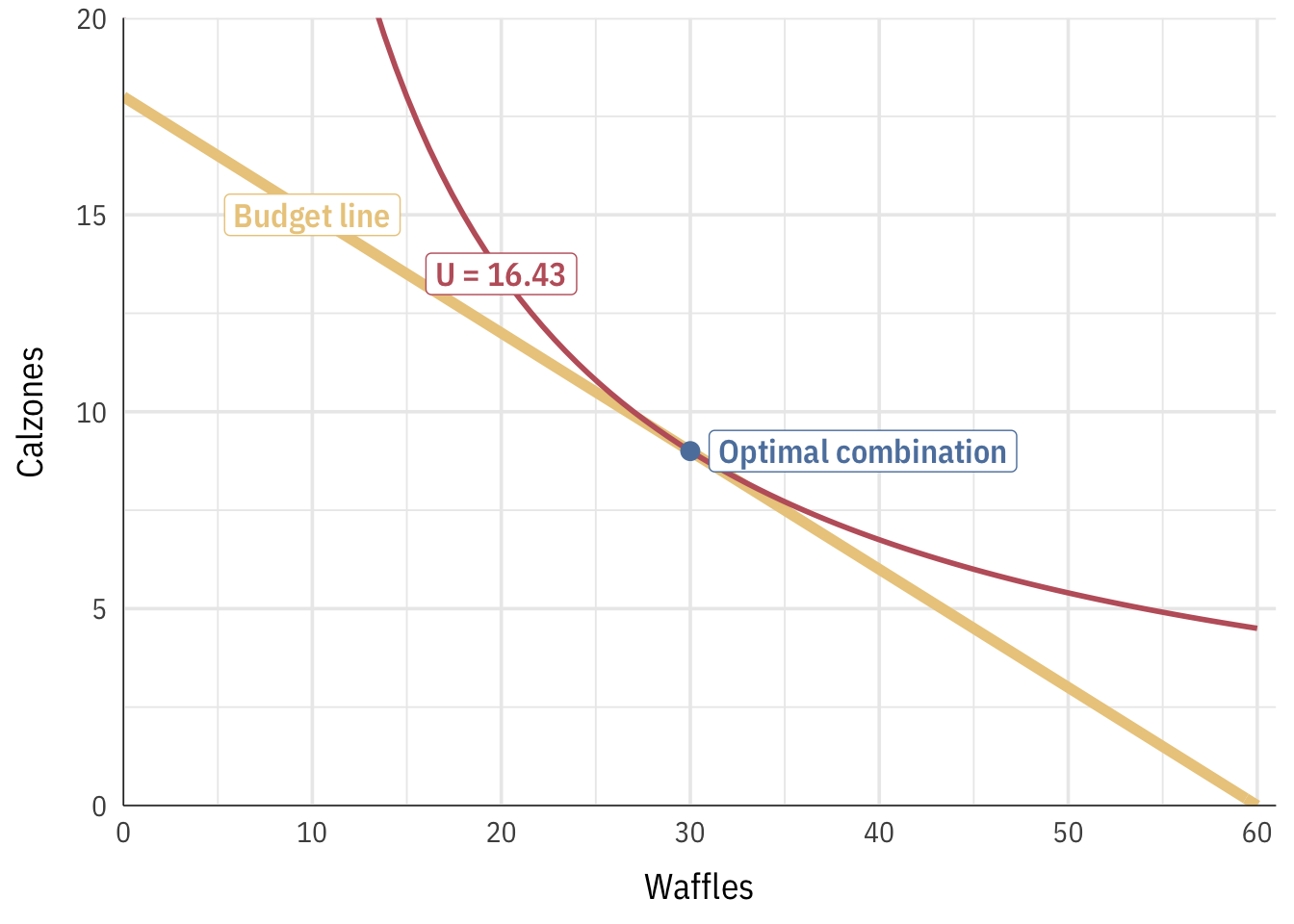# Utility maximization

The goal of maximizing utility is finding where the ideal meets reality, or where you can be the happiest given your constraints and scarcity. Often we talk about constraints with budgets, since working with dollars is easier than thinking about factory output or grades, like you saw in your ESPP reading.

When we talk about “reality” and “ideal” here, we mean two different things:

1. The feasible set (also known as the production possibility frontier or budget line): this represents the actual tradeoffs between two goods and the constraints on your choices. We’ve seen this with the airplane production functions (where there’s a tradeoff between workers and planes) and with Alexei’s decision to study (where there’s a tradeoff between hours of free time and his grade).

The slope of this line is known as the marginal rate of transformation (MRT), or the rate at which you can transform workers to planes or study hours to grades. The slope of this line is also the opportunity cost.

2. Indifference curves: these represent the theoretical tradeoff of two goods and your individual preferences. Each curve shows the combination of goods that produce the same level of utility.

The slope of this line is known as the marginal rate of substitution (MRS). It can be written a bunch of other ways:

$MRS = \frac{dy}{dx} = \frac{\Delta y}{\Delta x} = \frac{\text{Price}_x}{\text{Price}_y} = \frac{MU_x}{MU_y} = \frac{\partial u / \partial x}{\partial u / \partial y}$

We can find the optimum combination of goods (workers and planes, hours and grades, etc.) by combining the feasible set with indifference curves. With some algebra and calculus, we can find the combination of goods that maximizes utility, or find which indifference curve is tangent to the feasible set/budget line.

Don’t worry if you can’t remember calculus! You can use Wolfram Alpha to find derivatives automatically, or consult Khan Academy for a review of the power rule and calculating partial derivatives.

Here’s the general process for maximizing utility:

1. Figure out the feasible set (or budget line) and the marginal rate of transformation (MRT)
2. Use calculus and prices to figure out the marginal rate of substitution (MRS)
3. Set the budget line equal to the price-attuned MRS and use algebra to solve for $$x$$ and $$y$$

## Waffles and calzones

For this example, let’s pretend that you have to decide how many waffles and calzones to eat to make you the happiest possible. You have $20 to spend. What do you do?! Let’s write out the question more formally: Imagine that waffles (x) cost$1 and calzones (y) cost $2. You have a food budget of$20. Your utility function for waffles and calzones is $$u = xy$$.

Here’s how to figure this all out:

In this case our feasible set is not a production function—we aren’t limited by workers or time. Instead, we’re limited by our budget. We can only spend 20. If we spend all our money on calzones, we could buy 10 of them. If we spend all our money on waffles, we can buy 20 of them. We can plot all the combinations of waffles and calzones as a budget line:We can write this budget line as an equation following the $$y = mx + b$$ format, where $$m$$ is the slope and $$b$$ is the y-intercept. The slope here is the marginal rate of transformation (MRT). $y = -\frac{1}{2} x + 10$ ### 2. Use calculus and prices to figure out the marginal rate of substitution (MRS) We can afford every combination of waffles and calzones along the budget line, but we don’t know what the optimal mix of waffles and calzones is—that depends on how much we like the two foods, or our preferences. Our utility function is $$u = xy$$, which means that we multiply the quantity of waffles and calzones together to get our utility. That is, if we eat 10 waffles and 4 calzones, we’ll get 40 utils; if we eat 5 waffles and 14 calzones, we’ll get 70 utils; and so on. Indifference curves show all the combinations of two goods that provide the same utility. If we want to get 40 utils, we could eat 20 waffles and 2 calzones, 10 waffles and 4 calzones, 5 waffles and 8 calzones, etc. Each of those combinations provides 40 utils of happiness. We can calculate the combinations of waffles and calzones that lead to any amount of utility. In the chart below, I show three different indifference curves. Every point along the curve represents the combination of waffles and calzones that would lead to 10, 20, and 40 utils.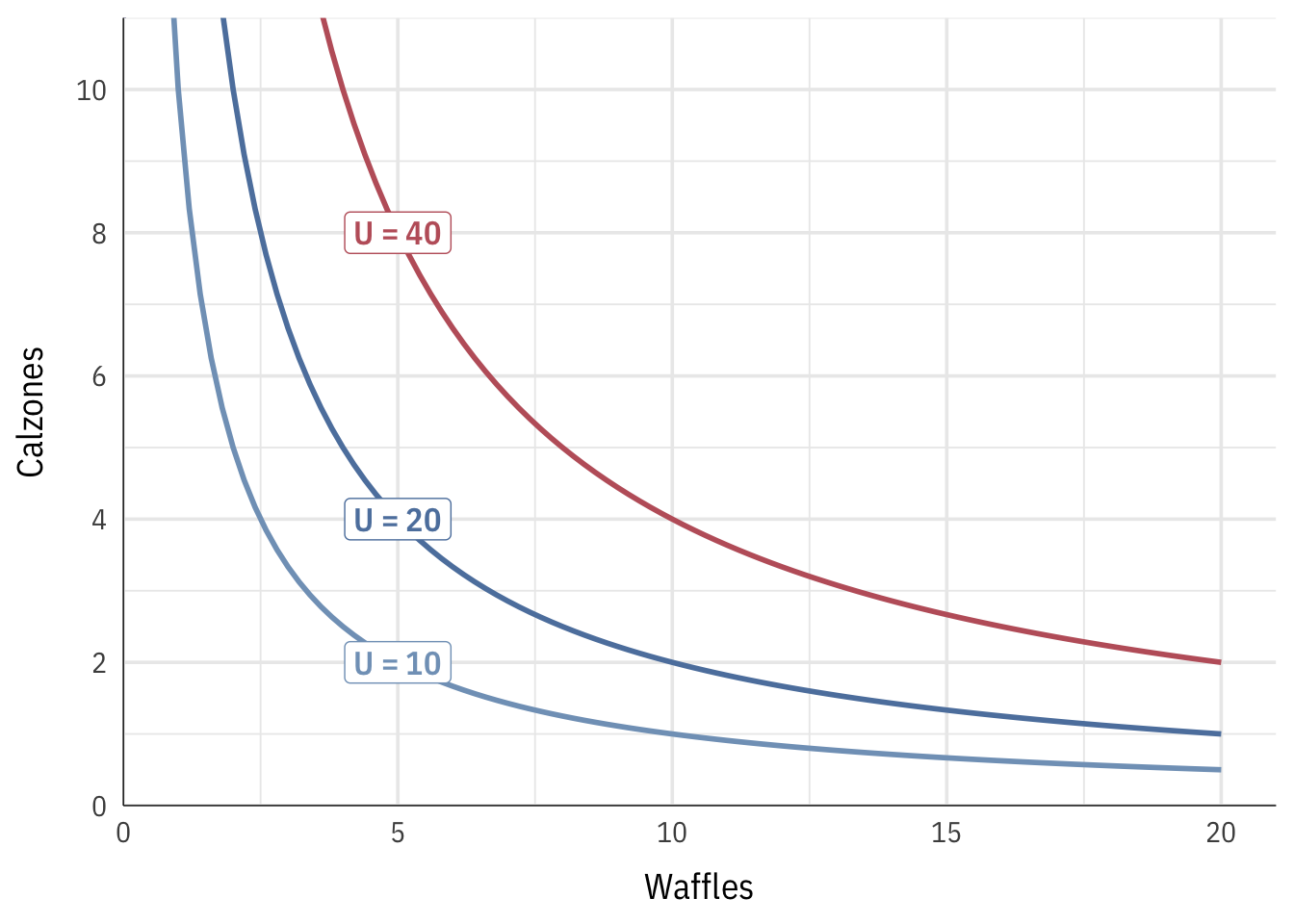Next, we can use this utility function to calculate the marginal rate of substitution or MRS, which is the slope of the curve at any given point. In calculus land, we find the slope of a function by calculating the first derivative. For simple one-variable functions like $$x^2$$, this involves moving the exponent down, multiplying it by the coefficient, and reducing the exponent by one. The first derivative of $$x^2$$ is $$2x$$. The derivative of $$2x^3$$ would be $$6x^2$$, and so on. When differentiating a two-variable function like $$xy$$, though, we can’t just follow the simple rule of moving an exponent down and subtracting one. Instead, we have to calculate partial derivatives—we find the derivative of just the $$x$$ part while holding $$y$$ constant and divide it by the derivative of just the $$y$$ part while holding $$x$$ constant. Don’t worry if that sounds complicated. The easiest way to do this is to go to Wolfram Alpha, type in the phrase “derivative xy” and see what it calculates for you. You’ll see two partial derivatives: $$\frac{\partial}{\partial x}$$ and $$\frac{\partial}{\partial y}$$. Make those two partial derivatives a ratio and you’ll have the derivative of the whole function: $$\frac{\partial / \partial x}{\partial / \partial y}$$. To make your life easier, I will occasionally provide you with the MRS in problem sets or exams (which I’ll calculate with Wolfram Alpha because who really wants to do calculus?!). In this case, where $$u = xy$$, the slope / first derivative / MRS is $$\frac{y}{x}$$. Next, we can add actual numbers to this MRS by finding the price-attuned version of the MRS. To do this, setting the MRS equal to the ratio of the prices of waffles and calzones, since MRS can also be written as $$\frac{\text{Price}_x}{\text{Price}_y}$$): $\frac{y}{x} = \frac{1}{2}$ We can use algebra to rearrange this formula so that it’s based on $$y$$: $y = \frac{1}{2} x$ That is our MRS given the prices that exist in the world. Phew. ### 3. Set the budget line equal to the price-attuned MRS and use algebra to solve for $$x$$ and $$y$$ Now that we have formulas for the MRT and the MRS, we can set them equal to each other to find where they are tangent to each other (i.e where their slopes are the same). Algebra time! \begin{aligned} \text{Price-attuned MRS} &= \text{Budget line} \\ \frac{1}{2} x &= -\frac{1}{2} x + 10 \\ x &= 10 \end{aligned} The optimal level of waffles is thus 10. We can plug that back into either the budget line or the MRT equation to figure out the optimal level of calzones: \begin{aligned} y &= -\frac{1}{2} x + 10 \\ y &= (-\frac{1}{2} \times 10) + 10 \\ y &= 5 \end{aligned} 5 calzones! The best combination food that maximizes our utility given our budget constraint and current prices is 10 waffles and 5 calzones. We can use our utility function to calculate how many utils we get from that level of consumption: $$u = xy$$, or 10 × 5, or 50. We can verify this combination graphically by plotting the budget line and indifference curve for 50 utils all at the same time:### tl;dr Desmos version You can avoid some of the math get the same answer by plotting these formulas at Desmos or with a graphing calculator: 1. Figure out the budget line or feasible set and plot it: $$y = -\frac{1}{2}x + 10$$ 2. Figure out the MRS ($$\frac{y}{x}$$), set it equal to the price ratio ($$\frac{1}{2}$$), and rearrange it so that it is in terms of y: $$y = \frac{1}{2} x$$. Plot that. 3. Notice how the two lines intersect at (10, 5). That’s the optimal point. 4. You can add an indifference curve by figuring out what level of utility you get when consuming 10 waffles and 5 calzones (10 × 5), and then plotting the utility function at that level. You don’t need to rearrange the formula or anything; Desmos is smart enough to figure it out: $$50 = xy$$. 5. If you did it right, you should get an indifference curve tangent to the budget line at (10, 5).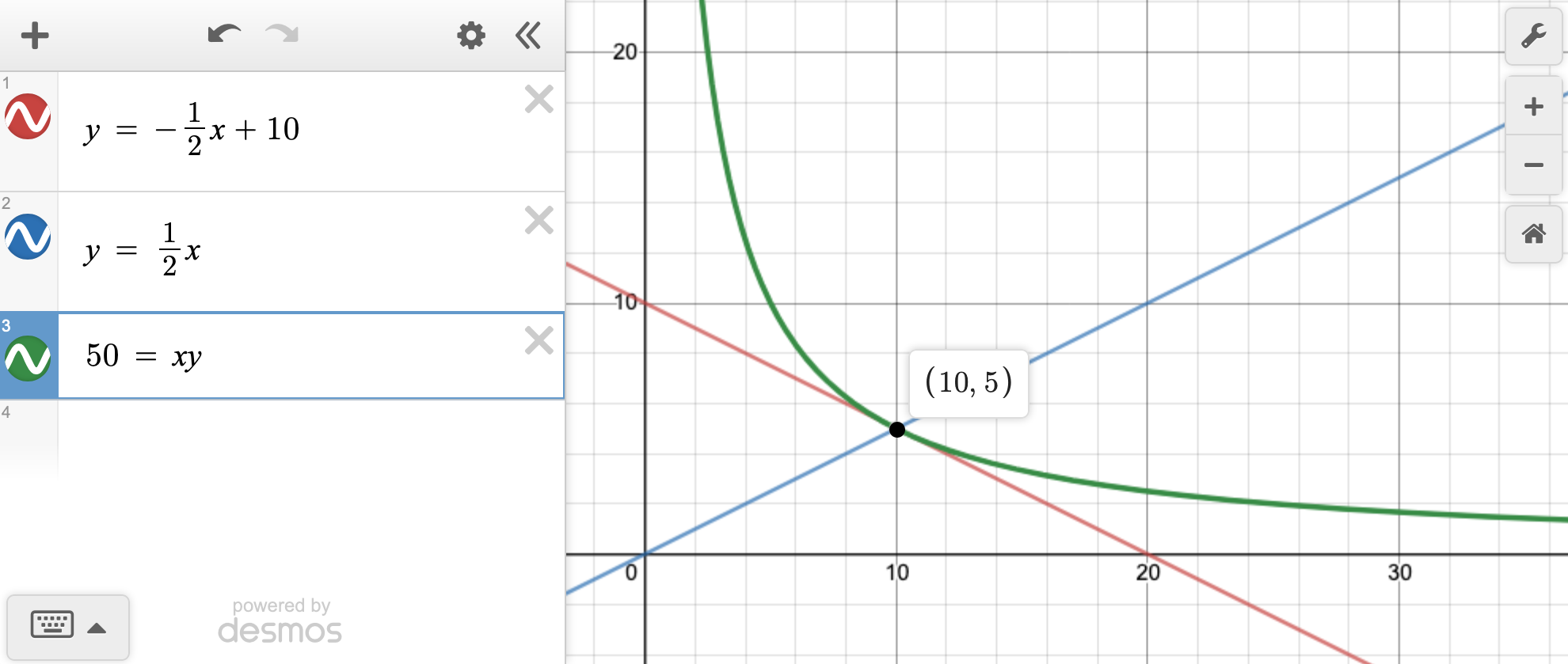## Waffles and calzones with different values To show some additional examples of how to do this, here are a few different versions of the same question but with different prices, budgets, and utility functions. ### Example 1 Here are the constraints and preferences: • Waffles ($$x$$) =3
• Calzones ($$y$$) = $6 • Budget =$60
• Utility = $$u = x^2 y$$

Step 1: Find the feasible set/budget line

If we spent 100% of the budget on waffles, we could get 20 waffles; if we spent it all on calzones, we could get 10. That creates this budget line:

$y = -\frac{1}{2}x + 10$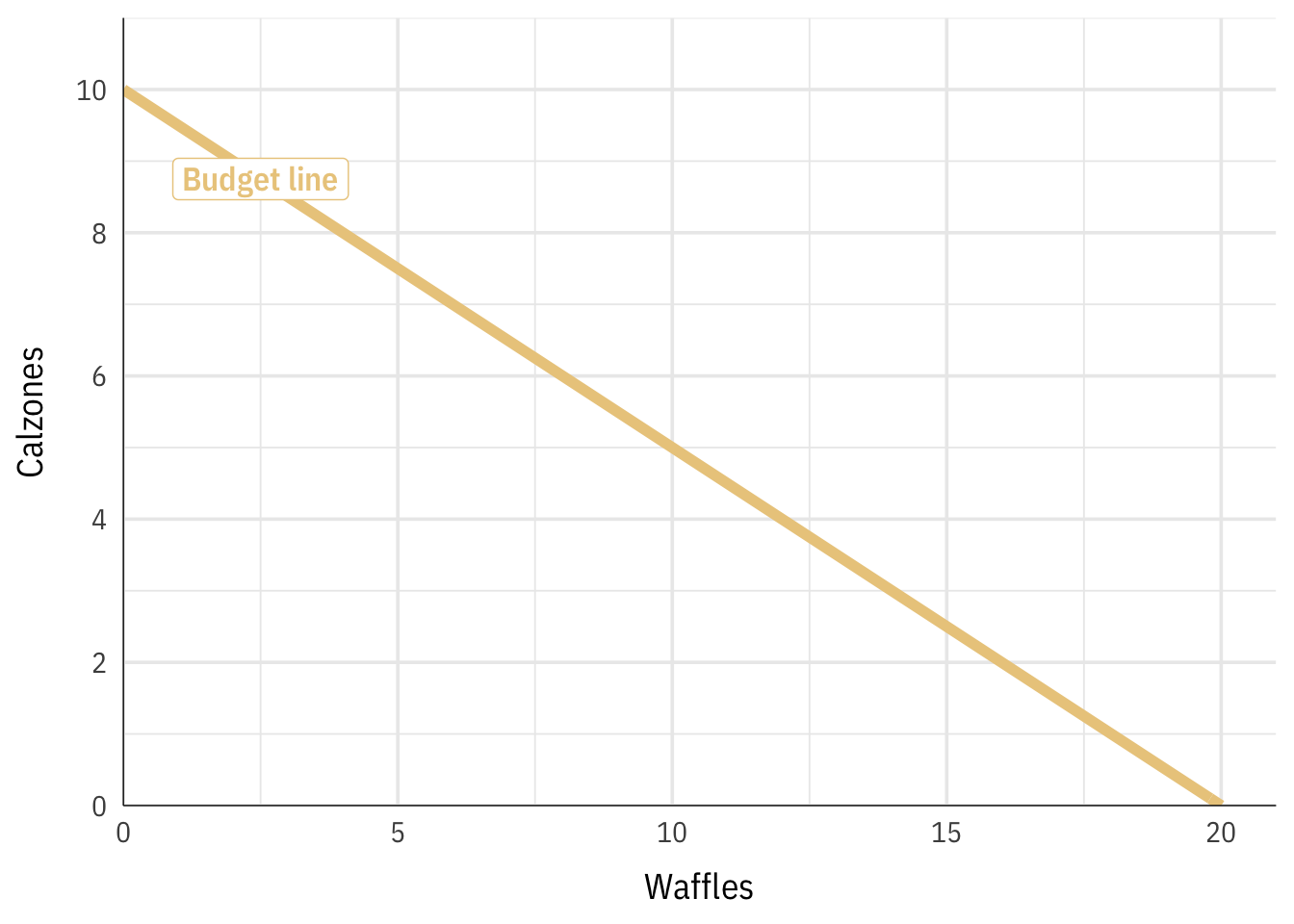Step 2: Find the MRS and add prices to it

Our utility function is $$u = x^2 y$$. To find the slope of this equation (or the MRS), we need to find the derivative, and because there are two variables, we need to find the partial derivative of the $$x$$ part and the partial derivative of the $$y$$ part. Wolfram Alpha can do this for us (type “derivative x^2 y”):

• $$\frac{\partial}{\partial x} = 2xy$$
• $$\frac{\partial}{\partial y} = x^2$$

If we make these a ratio ($$\frac{\partial / \partial x}{\partial / \partial y}$$), we end up with the following formula:

\begin{aligned} \frac{\partial / \partial x}{\partial / \partial y} &= \frac{2xy}{x^2} \\ &= \frac{2y}{x} \end{aligned}

The slope of the indifference curve at any combination of $$x$$ and $$y$$ is thus $$\frac{2y}{x}$$.

Next, we need to build the prices into the formula by setting the MRS equal to the ratio of prices ($$\frac{\text{Price}_x}{\text{Price}_y}$$) and rearranging so the formula starts with $$y =$$:

\begin{aligned} \frac{2y}{x} &= \frac{3}{6} \\ 12y &= 3x \\ y &= \frac{1}{4}x \end{aligned}

Our price-attuned MRS is thus $$y = \frac{1}{4}x$$.

Step 3: Set the budget line equal to the MRS and solve for $$x$$ and $$y$$

Finally we can set the two equations equal to each other to find where they cross (or just put them in Desmos):

\begin{aligned} -\frac{1}{2}x + 10 &= \frac{1}{4}x \\ 10 &= \frac{3x}{4} \\ 40 &= 3x \\ 13.333 \text{ (or } \frac{40}{3} \text{)} &= x \end{aligned} The ideal number of waffles is 13.33. Let’s plug that back into the budget line to see how many calzones we can get if we get that many waffles:

\begin{aligned} y &= -\frac{1}{2} x + 10 \\ y &= -\frac{1}{2} \times 13.333 + 10 \\ y &= 3.333 \text{ (or } \frac{10}{3} \text{)} \end{aligned}

We can get 3.33 calzones. The best combination of waffles and calzones is thus 13.33 and 3.33. We can calculate the utility we’d get from that by plugging these values into the utility function:

\begin{aligned} u &= x^2 y \\ u &= 13.333^2 \times 3.333 \text{ (or } \frac{40}{3}^2 \times \frac{10}{3} \text{)} \\ u &= 592.5864 \end{aligned}

We’d get 592.5864 utils from 13.333 waffles and 3.333 calzones. Here’s everything all together: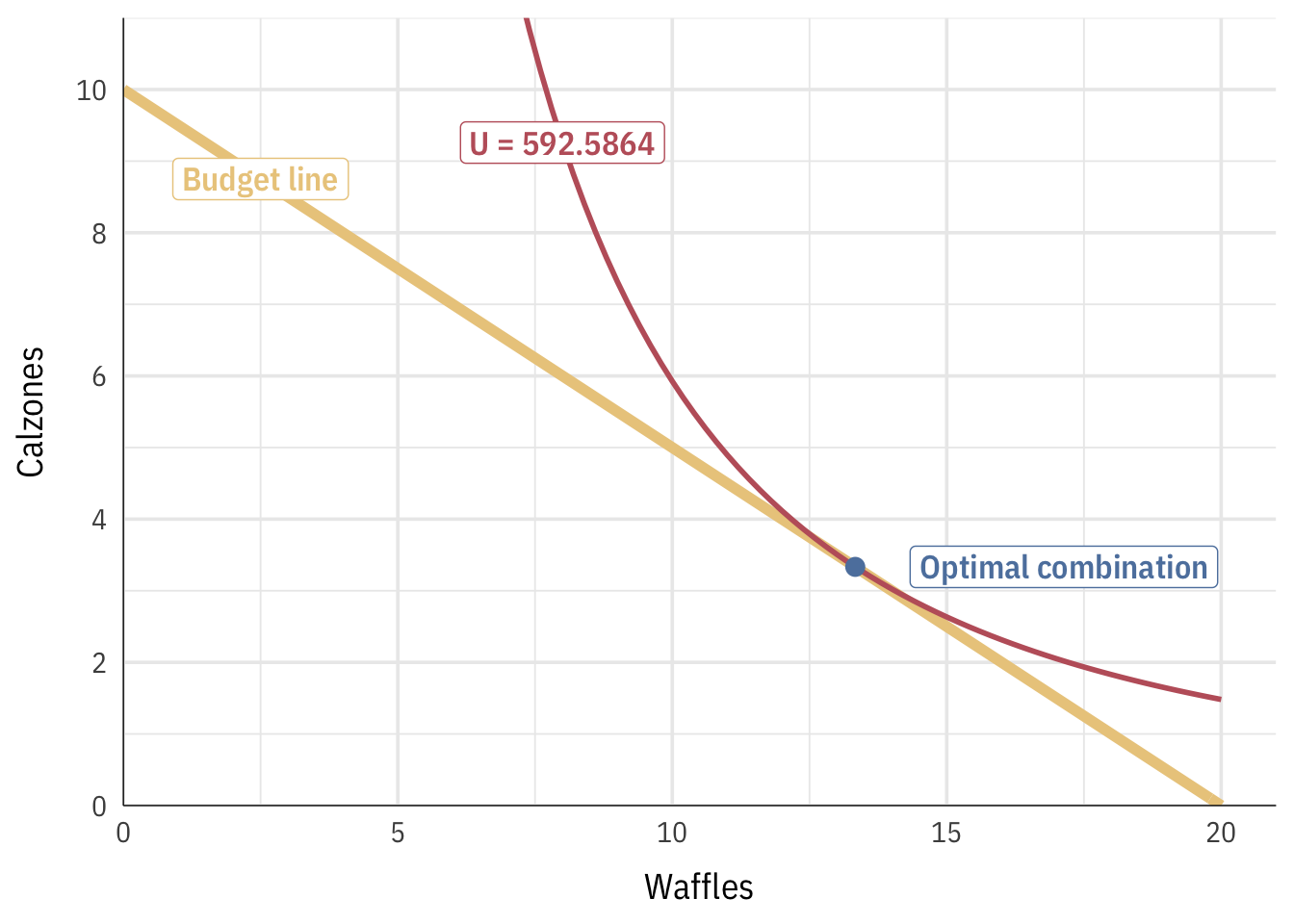### Example 2

• Waffles ($$x$$) = $3 • Calzones ($$y$$) =$1.50
• Budget = 45 • Utility = $$u = x^2 \times \frac{1}{4}y$$ Step 1: Find the feasible set/budget line If we spent 100% of the budget on waffles, we could get 15 waffles; if we spent it all on calzones, we could get 30. That creates this budget line: $y = -2x + 30$Step 2: Find the MRS and add prices to it Our utility function is $$u = x^2 \times \frac{1}{4}y$$. To find the slope of this equation (or the MRS), we need to find the derivative, and because there are two variables, we need to find the partial derivative of the $$x$$ part and the partial derivative of the $$y$$ part. Wolfram Alpha can do this for us (type “derivative x^2 * 1/4y”): • $$\frac{\partial}{\partial x} = \frac{xy}{2}$$ • $$\frac{\partial}{\partial y} = \frac{x^2}{4}$$ If we make these a ratio ($$\frac{\partial / \partial x}{\partial / \partial y}$$), we end up with the following formula: \begin{aligned} \frac{\partial / \partial x}{\partial / \partial y} &= \frac{xy / 2}{x^2 / 4} \\ &= \frac{2y}{x} \end{aligned} The slope of the indifference curve at any combination of $$x$$ and $$y$$ is thus $$\frac{2y}{x}$$. Next, we need to build the prices into the formula by setting the MRS equal to the ratio of prices ($$\frac{\text{Price}_x}{\text{Price}_y}$$) and rearranging so the formula starts with $$y =$$: \begin{aligned} \frac{2y}{x} &= \frac{3}{1.5} \\ 3y &= 3x \\ y &= x \end{aligned} Our price-attuned MRS is thus $$y = x$$ (that’s nice and easy!). Step 3: Set the budget line equal to the MRS and solve for $$x$$ and $$y$$ Finally we can set the two equations equal to each other to find where they cross (or just put them in Desmos): \begin{aligned} -2x + 30 &= x \\ 30 &= 3x \\ 10 &= x \end{aligned} The ideal number of waffles is 10. Let’s plug that back into the budget line to see how many calzones we can get if we get that many waffles: \begin{aligned} y &= -2x + 30 \\ y &= 2 \times 10 + 30 \\ y &= 10 \end{aligned} We can get 10 calzones. The best combination of waffles and calzones is thus 10 and 10. We can calculate the utility we’d get from that by plugging these values into the utility function: \begin{aligned} u &= x^2 \times \frac{1}{4}y \\ u &= 10^2 \times (0.25 \times 10) \\ u &= 250 \end{aligned} We’d get 250 utils from 10 waffles and 10 calzones. Here’s everything all together:### Example 3 Let’s do one more: • Waffles ($$x$$) =1.50
• Calzones ($$y$$) = $5 • Budget =$90
• Utility = $$u = \sqrt{xy}$$

Step 1: Find the feasible set/budget line

If we spent 100% of the budget on waffles, we could get 60 waffles; if we spent it all on calzones, we could get 18. That creates this budget line:

$y = -\frac{3}{10}x + 18$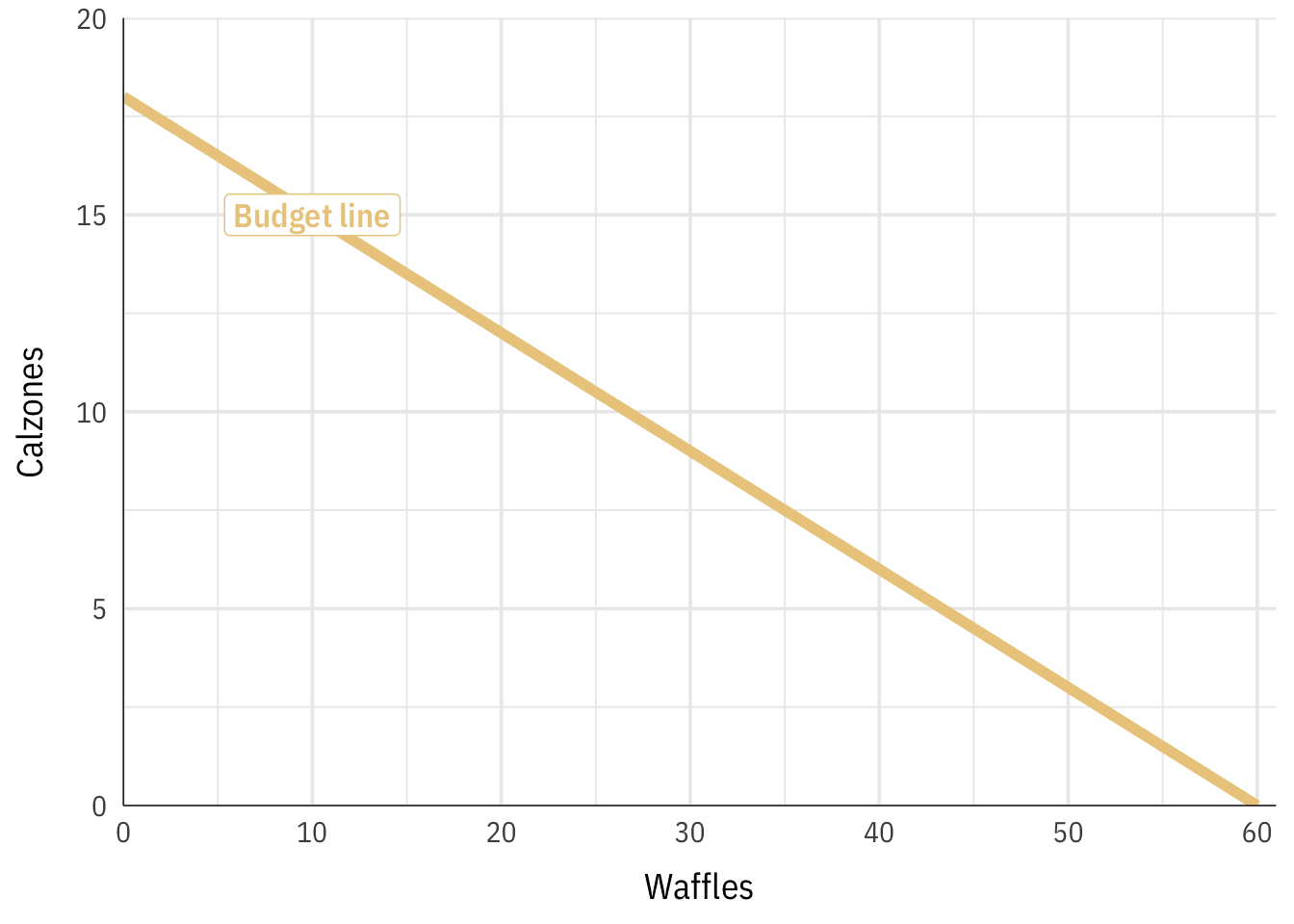Step 2: Find the MRS and add prices to it

Our utility function is $$u = \sqrt{x}{y}$$. To find the slope of this equation (or the MRS), we need to find the derivative, and because there are two variables, we need to find the partial derivative of the $$x$$ part and the partial derivative of the $$y$$ part. Wolfram Alpha can do this for us (type “derivative sqrt(xy)”):

• $$\frac{\partial}{\partial x} = \frac{y}{2 \sqrt{xy}}$$
• $$\frac{\partial}{\partial y} = \frac{x}{2 \sqrt{xy}}$$

If we make these a ratio ($$\frac{\partial / \partial x}{\partial / \partial y}$$), we end up with the following formula:

\begin{aligned} \frac{\partial / \partial x}{\partial / \partial y} &= \frac{y / 2 \sqrt{xy}}{x / 2 \sqrt{xy}} \\ &= \frac{y}{x} \end{aligned}

The slope of the indifference curve at any combination of $$x$$ and $$y$$ is thus $$\frac{y}{x}$$.

Next, we need to build the prices into the formula by setting the MRS equal to the ratio of prices ($$\frac{\text{Price}_x}{\text{Price}_y}$$) and rearranging so the formula starts with $$y =$$:

\begin{aligned} \frac{y}{x} &= \frac{1.5}{5} \\ 5y &= 1.5x \\ y &= 0.3x \text{ (or } \frac{3}{10}x \text{)} \end{aligned}

Our price-attuned MRS is thus $$y = 0.3x$$.

Step 3: Set the budget line equal to the MRS and solve for $$x$$ and $$y$$

Finally we can set the two equations equal to each other to find where they cross (or just put them in Desmos):

\begin{aligned} -\frac{3}{10}x + 18 &= \frac{3}{10}x \\ 18 &= \frac{6}{10}x \\ 30 &= x \end{aligned}

The ideal number of waffles is 30. Let’s plug that back into the budget line to see how many calzones we can get if we get that many waffles:

\begin{aligned} y &= -\frac{3}{10}x + 18 \\ y &= -\frac{3}{10} \times 30 + 18 \\ y &= 9 \end{aligned}

We can get 9 calzones. The best combination of waffles and calzones is thus 30 and 9. We can calculate the utility we’d get from that by plugging these values into the utility function:

\begin{aligned} u &= \sqrt{xy} \\ u &= \sqrt{30 \times 9} \\ u &= 16.43 \end{aligned}

We’d get 16.43 utils from 30 waffles and 9 calzones. Here’s everything all together: